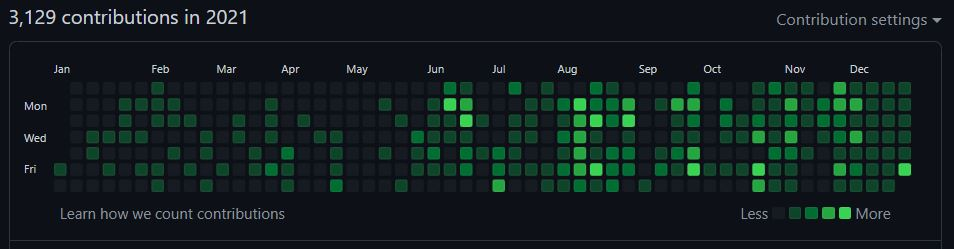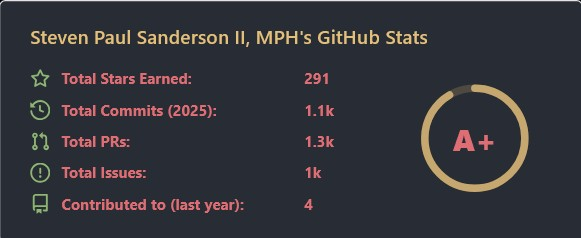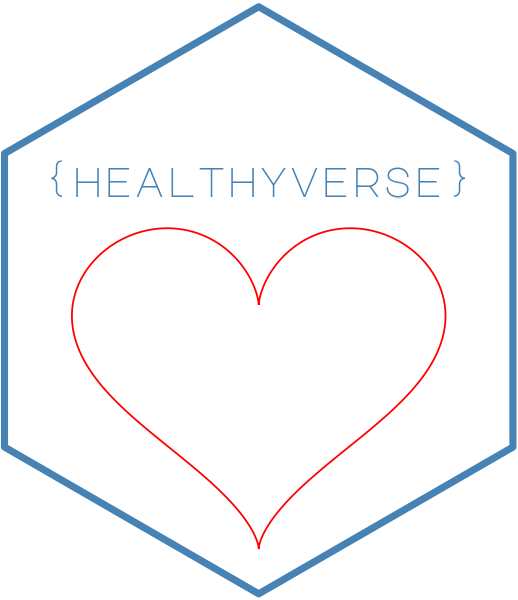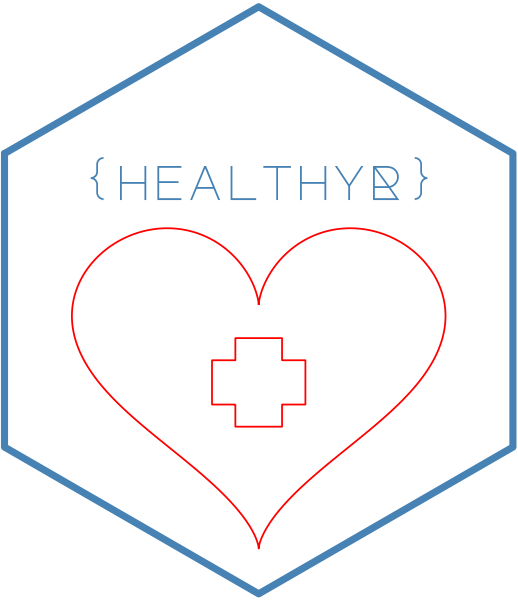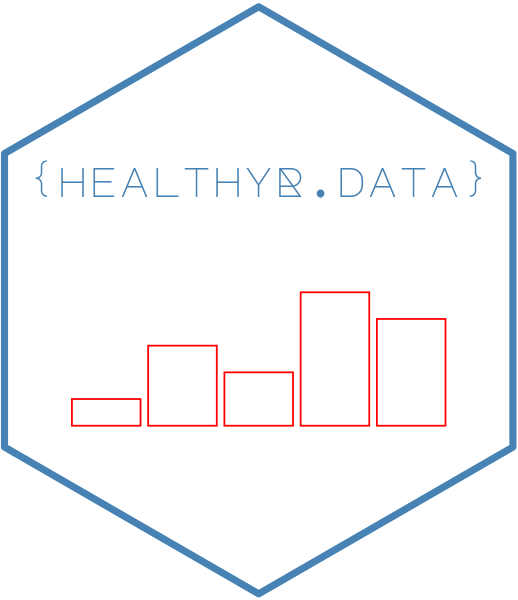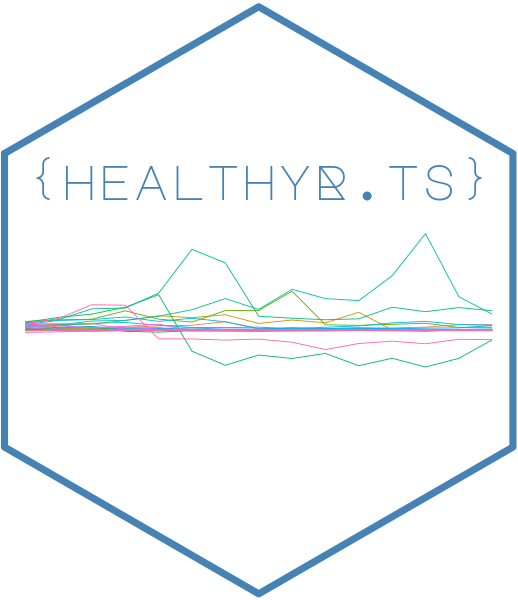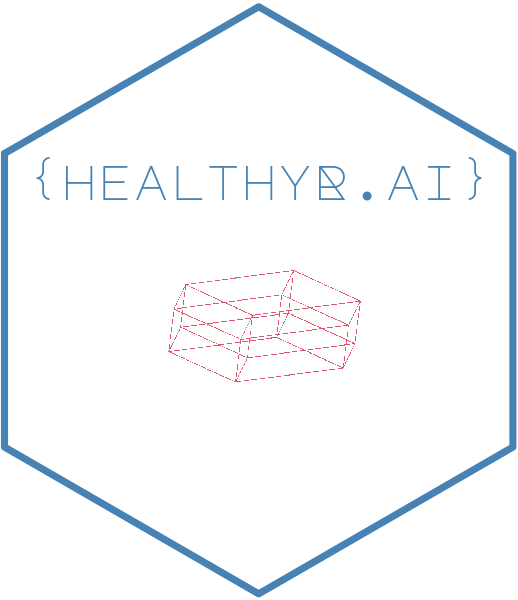2021 A Year in Review

The year 2021 was a big year for me. I did a lot of coding, a lot more than I typically do. The biggest push came personally in my ongoing development of my R packages that are in the healthyverse. To use the healthyverse simply do so in the familiar fashion:

install.packages("healthyverse")
library(healthyverse)

Here are links to all of the packages:

In order to start looking at some of the data that pertains to 2021 lets first get the data from the CRAN logs. Since I do this daily already, I can simply use the rds file I already have. I am going to go through the motions though, in case others might want to do something similar. The functions I am using to get the data can be found here

Now lets get that data!

library(tidyverse)
library(lubridate)
source("01_scripts/get_data_functions.R")
source("01_scripts/data_manipulation_functions.R")
source("01_scripts/mapping_functions.R")

get_cran_data()
get_package_release_data()
csv_to_rds()

Ok now that we have our data, lets ensure that we are only using the year 2021. We can do this by filtering out data by time with the timetk package.

Now lets filter our data below, some pre-processing may need to take place.

library(timetk)
filter_by_time(
.date_var = date,
.start_date = "2021",
.end_date = "2021"
)

glimpse(data_tbl)
## Rows: 24,887
## Columns: 11
## $date <date> 2021-01-01, 2021-01-01, 2021-01-01, 2021-01-01, 2021-01-01,… ##$ time      <Period> 3H 41M 37S, 3H 42M 2S, 10H 1M 57S, 10H 1M 57S, 10H 1M 57S…
## $date_time <dttm> 2021-01-01 03:41:37, 2021-01-01 03:42:02, 2021-01-01 10:01:… ##$ size      <int> 2910045, 5668471, 533, 5669257, 81738, 5668465, 530, 14701, …
## $r_version <chr> "4.0.3", "3.6.0", NA, NA, NA, NA, NA, NA, NA, NA, NA, NA, NA… ##$ r_arch    <chr> "x86_64", "x86_64", NA, NA, NA, NA, NA, NA, NA, NA, NA, NA, …
## $r_os <chr> "mingw32", "mingw32", NA, NA, NA, NA, NA, NA, NA, NA, NA, NA… ##$ package   <chr> "healthyR", "healthyR.data", "healthyR.data", "healthyR.data…
## $version <chr> "0.1.1", "1.0.0", "1.0.0", "1.0.0", "1.0.0", "1.0.0", "1.0.0… ##$ country   <chr> "GH", "GH", "US", "US", "US", "US", "US", "US", "US", "US", …
## \$ ip_id     <int> 220, 220, 8154, 8154, 8154, 8154, 8154, 8154, 8154, 8154, 81…

Now that we have our data, we have it for the year 2021 only with a start date of 2021-01-01 and an end date of 2021-12-31.

# Package Information

The first thing we will do is look at how many downloads there were for each pacakge and it’s version.

library(knitr)

data_tbl %>%
count(package, version) %>%
pivot_wider(
id_cols       = version
, names_from  = package
, values_from = n
, values_fill = 0
) %>%
arrange(version) %>%
kable()
version healthyR healthyR.ai healthyR.data healthyR.ts healthyverse
0.0.1 0 271 0 0 0
0.0.2 0 1519 0 0 0
0.0.3 0 281 0 0 0
0.0.4 0 324 0 0 0
0.1.0 89 0 0 386 0
0.1.1 813 0 0 1867 0
0.1.2 1388 0 0 907 0
0.1.3 232 0 0 1027 0
0.1.4 280 0 0 586 0
0.1.5 931 0 0 422 0
0.1.6 2083 0 0 165 0
0.1.7 0 0 0 314 0
1.0.0 0 0 2003 0 2199
1.0.1 0 0 4854 0 1946

Now lets see how many total downloads for the year there were for each package.

data_tbl %>%
count(package) %>%
kable()
healthyR 5816
healthyR.ai 2395
healthyR.data 6857
healthyR.ts 5674
healthyverse 4145
data_tbl %>%
select(package, version) %>%
group_by(package) %>%
distinct() %>%
mutate(release_count = n()) %>%
ungroup() %>%
select(package, release_count) %>%
distinct() %>%
set_names("Package", "Number of Releases") %>%
kable()
Package Number of Releases
healthyR 7
healthyR.data 2
healthyR.ts 8
healthyverse 2
healthyR.ai 4
total_number_of_releases <- data_tbl %>%
select(package, version) %>%
group_by(package) %>%
distinct() %>%
mutate(release_count = n()) %>%
ungroup() %>%
select(package, release_count) %>%
distinct() %>%
summarise(total = sum(release_count, na.rm = TRUE))

So all in all there was a total of 24,887 downloads of all the healthyverse packages in 2021. There were in total 23 package releases as well.

# Graphs

Now lets graph the data out!

data_tbl %>%
count(package, version) %>%
ggplot(aes(x = version, y = n, alpha = 0.382)) +
geom_col(aes(group = package, fill = package)) +
facet_wrap(package ~., ncol = 2, scales = "free") +
scale_y_continuous(labels = scales::label_number(big.mark = ",")) +
theme_minimal() +
theme(legend.position = "bottom") +
labs(
subtitle = "Faceted by Package",
x = "Version",
fill = "Package"
)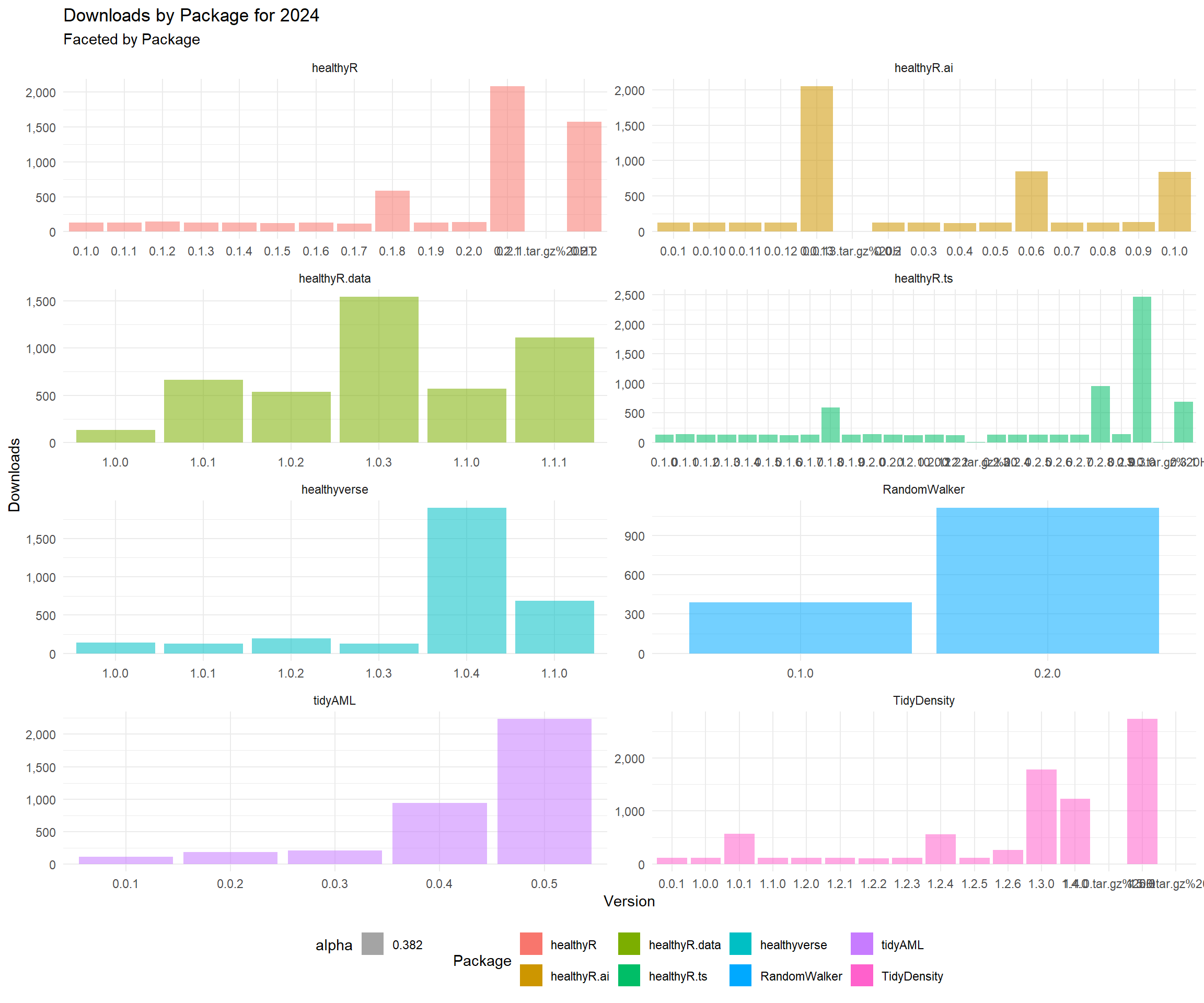data_tbl %>%
count(package, version) %>%
group_by(package) %>%
mutate(record = row_number()) %>%
ungroup() %>%
geom_col(aes(group = package, fill = package)) +
facet_wrap(package ~., ncol = 2, scales = "free") +
scale_y_continuous(labels = scales::label_number(big.mark = ",")) +
theme_minimal() +
theme(legend.position = "bottom") +
labs(
subtitle = "Faceted by Package",
x = "Relase Number",
fill = "Package"
)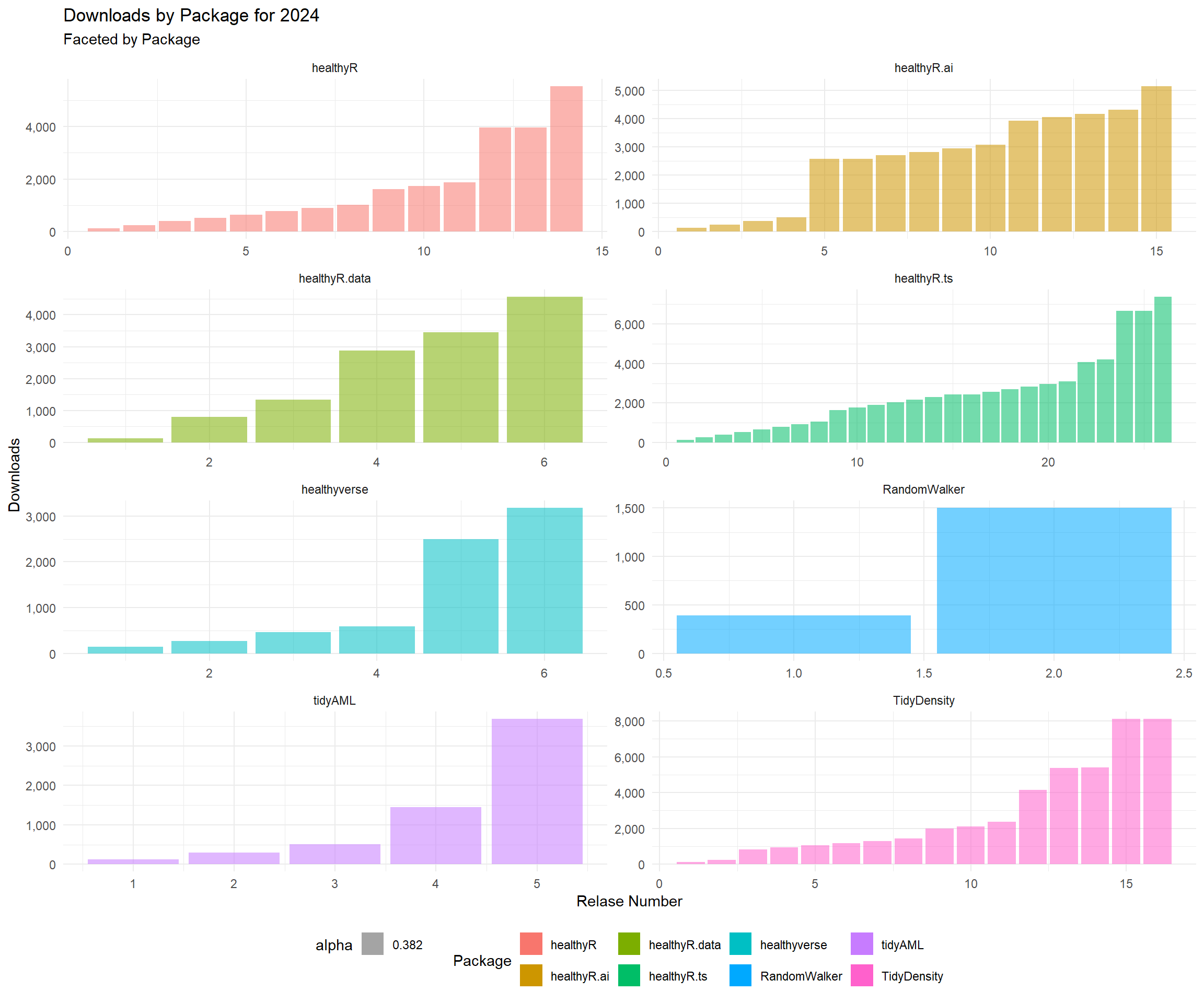data_tbl %>%
count(package, version) %>%
group_by(package) %>%
mutate(record = row_number()) %>%
ungroup() %>%
geom_line(aes(color = package, group = package), size = 1) +
scale_y_continuous(labels = scales::label_number(big.mark = ",")) +
theme_minimal() +
theme(legend.position = "bottom") +
labs(
subtitle = "Colored by Package",
x = "Release Number",
color = "Package"
)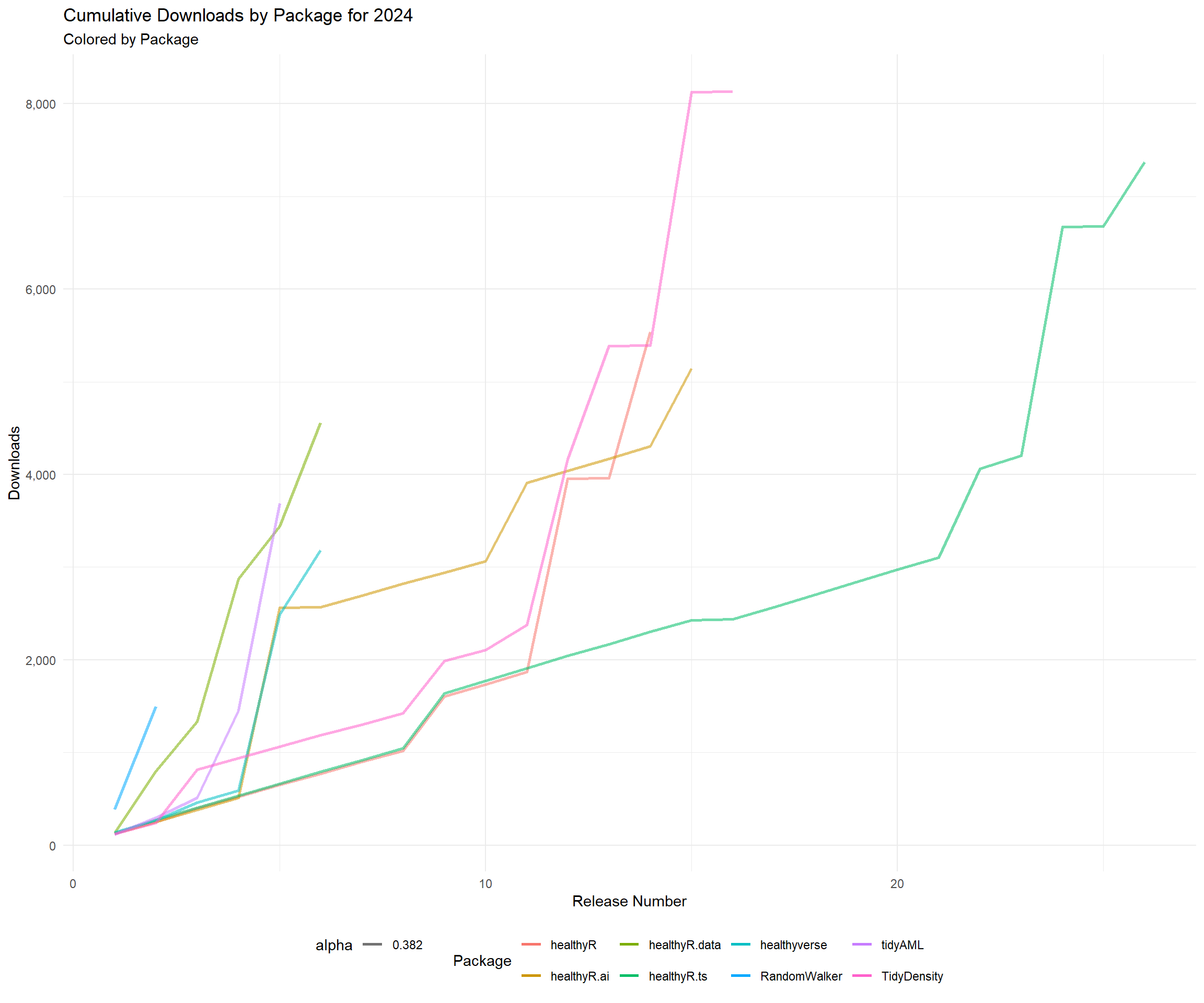# Time Series Graphs

Now lets get some time-series graphs.

library(healthyR.ts)

data_tbl %>%
summarise_by_time(.date_var = date, n = n()) %>%
ts_calendar_heatmap_plot(.date_col = date, .value_col = n, .interactive = FALSE)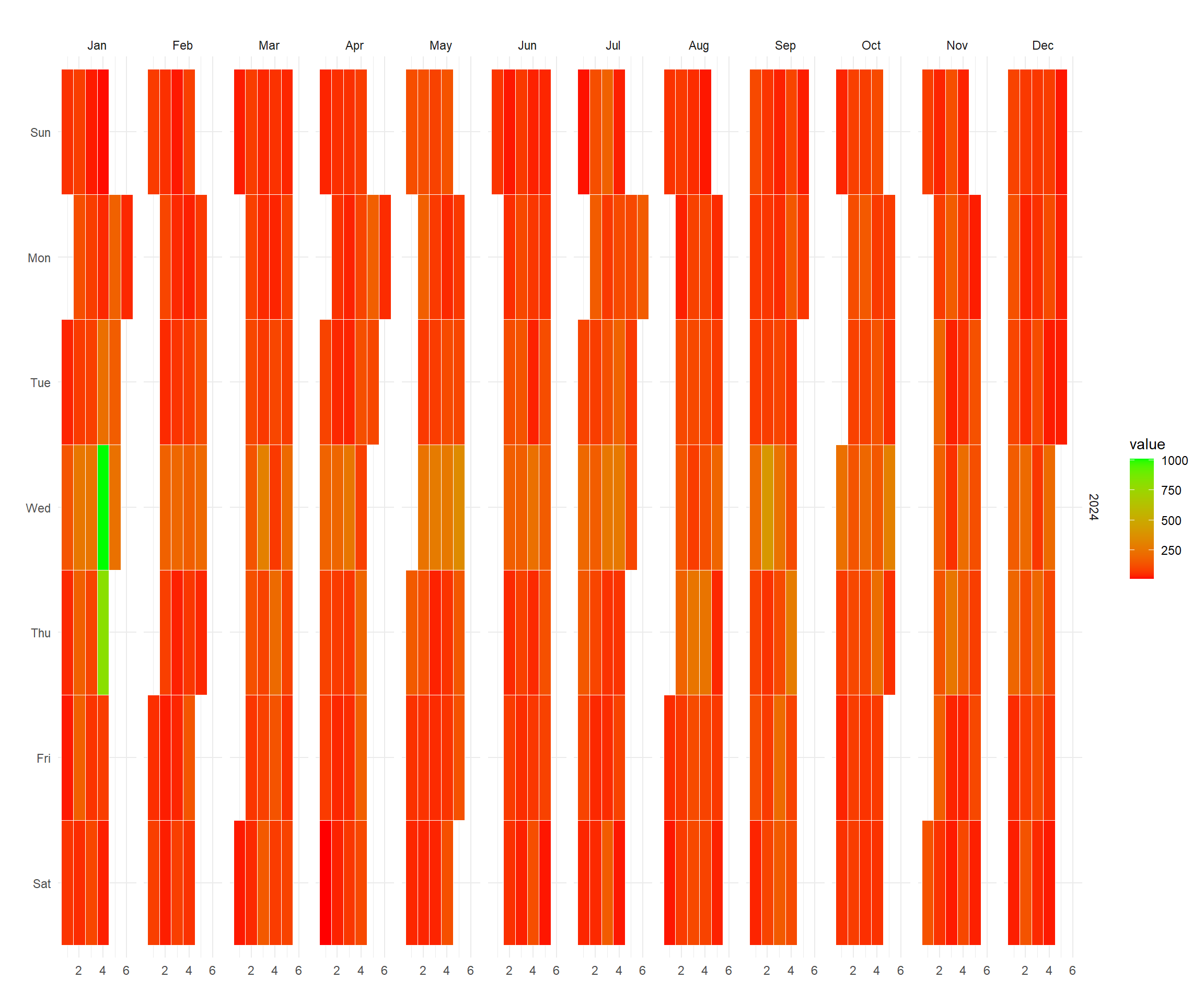data_tbl %>%
ggplot(aes(date, log1p(value))) +
geom_point(aes(group = package, color = package), size = 1) +
geom_smooth(method = "loess", color = "black",  se = FALSE) +
geom_vline(
data = pkg_tbl
, aes(xintercept = as.Date(date))
, color = "red"
, lwd = 1
, lty = "solid"
) +
facet_wrap(package ~., ncol = 2, scales = "free_x") +
theme_minimal() +
labs(
subtitle = "Vertical lines represent release dates",
x = "Date",
y = "log1p(Counts)",
color = "Package"
) +
theme(legend.position = "bottom")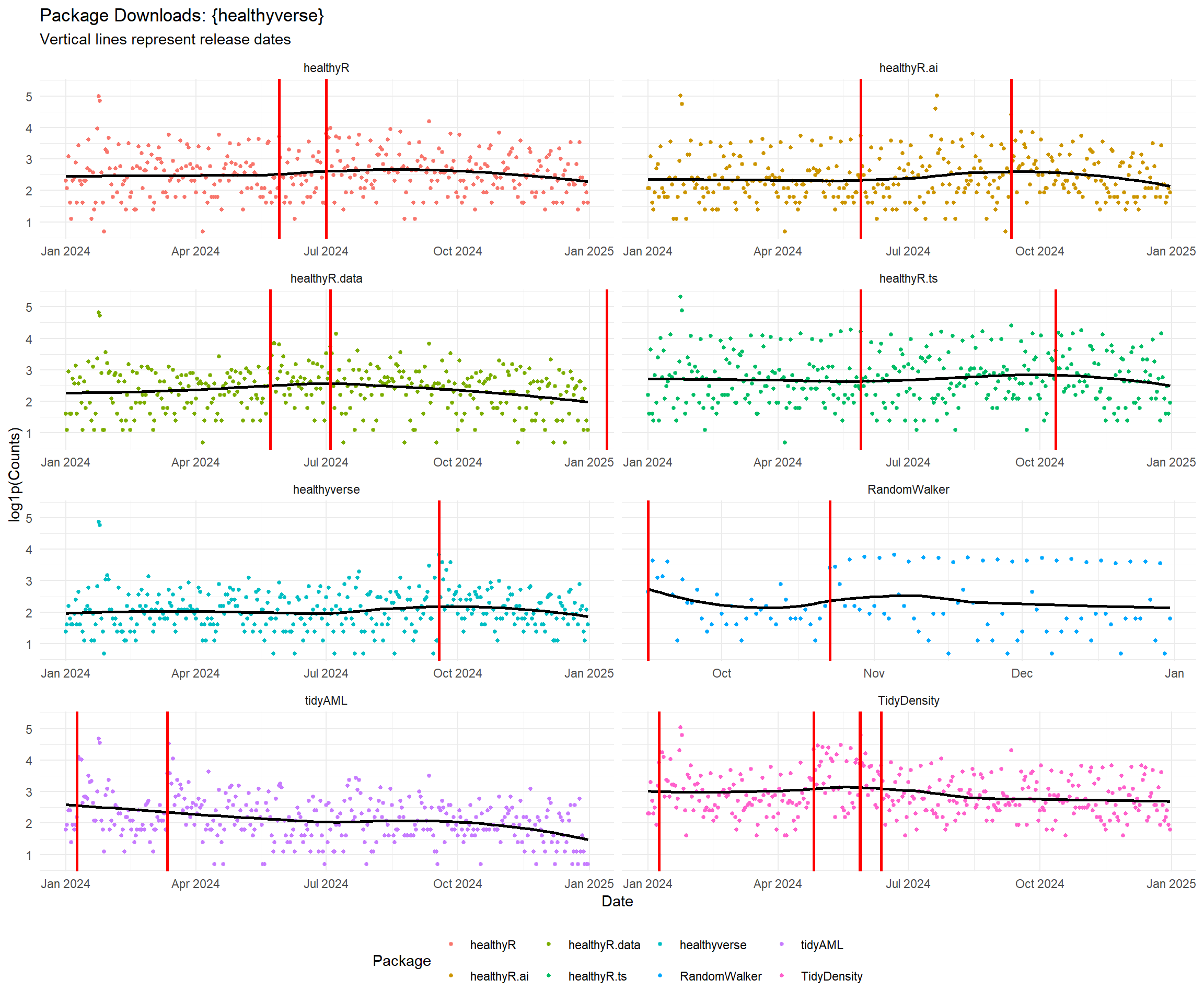data_tbl %>%
rename(Actual = value) %>%
tk_augment_differences(.value = Actual, .differences = 1) %>%
tk_augment_differences(.value = Actual, .differences = 2) %>%
rename(velocity = contains("_diff1")) %>%
rename(acceleration = contains("_diff2")) %>%
pivot_longer(-date) %>%
mutate(name = str_to_title(name)) %>%
mutate(name = as_factor(name)) %>%
ggplot(aes(x = date, y = log1p(value), group = name)) +
geom_point(alpha = .2) +
geom_vline(
data = pkg_tbl
, aes(xintercept = as.Date(date), color = package)
, lwd = 1
, lty = "solid"
) +
facet_wrap(name ~ ., ncol = 1, scale = "free") +
theme_minimal() +
labs(
subtitle = "Vertical Lines Indicate a CRAN Release date for a package.",
x = "Date",
y = "",
color = ""
) +
theme(legend.position = "bottom")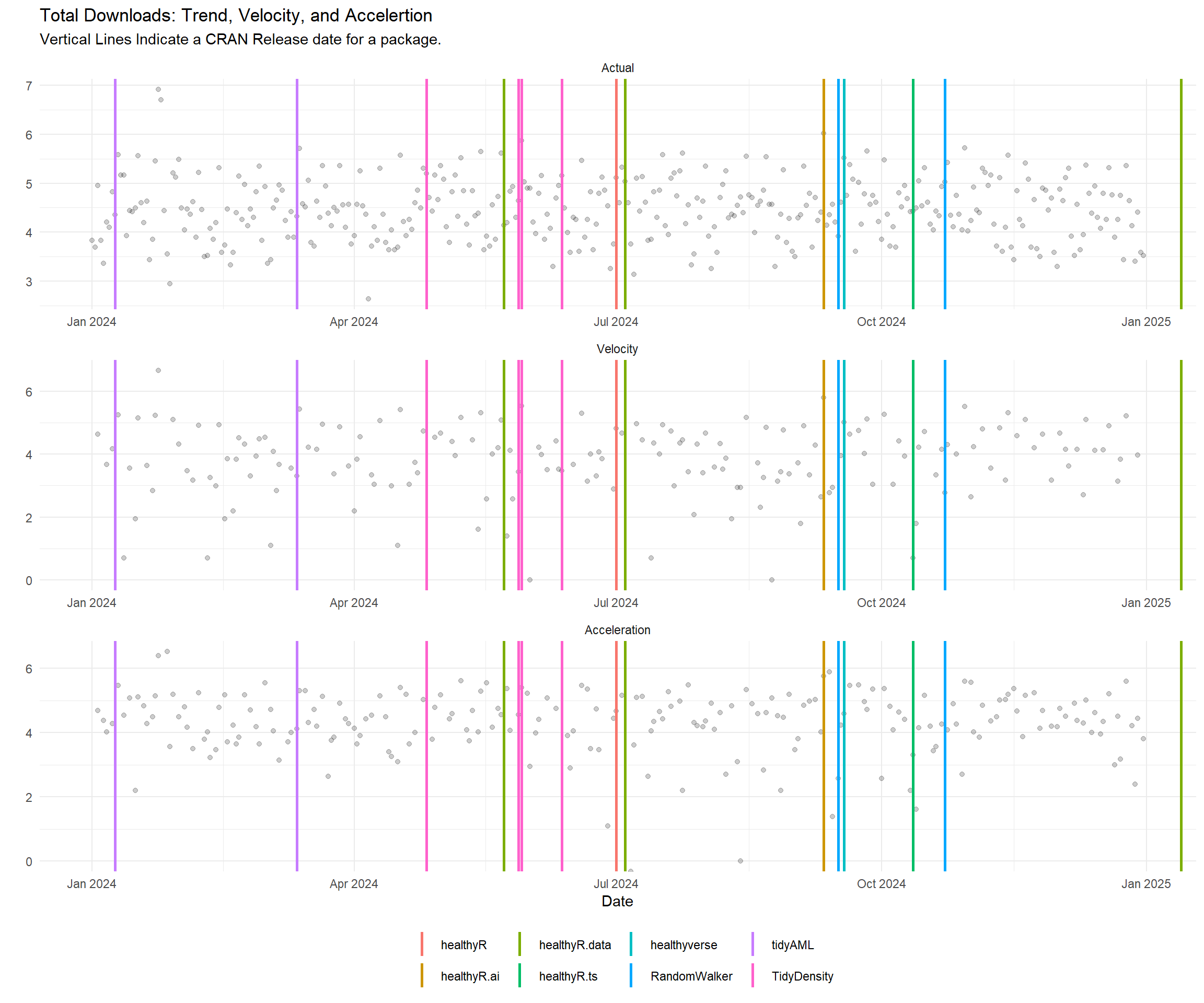data_tbl %>%
plot_seasonal_diagnostics(
.date_var = date,
.value = log1p(value),
.interactive = FALSE
) +
theme_minimal() +
labs(
title = "Seasonal Diagnostics",
subtitle = "Values are log1p"
)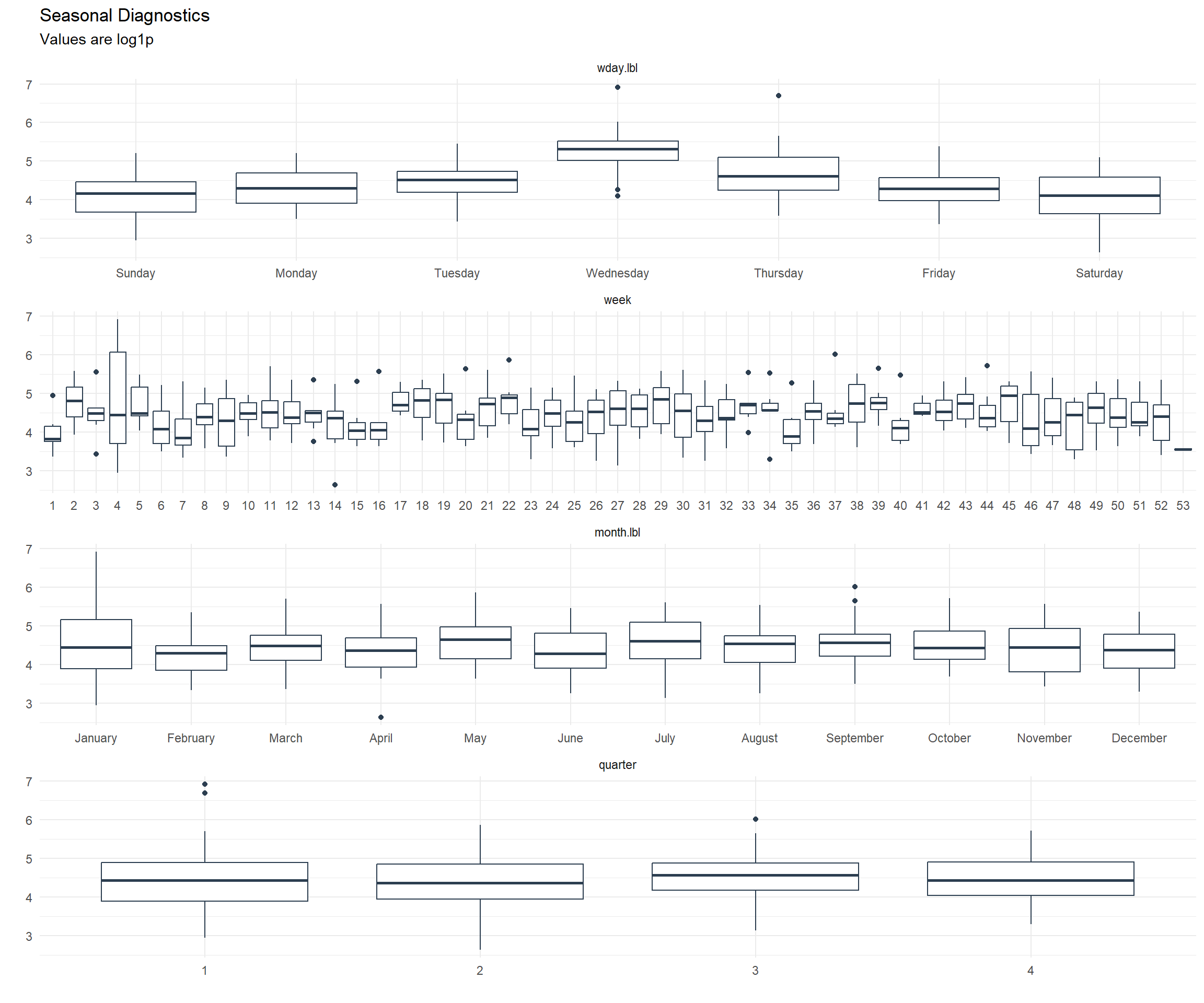data_tbl %>%
plot_stl_diagnostics(
.date_var = date,
.value = log1p(value),
.interactive = FALSE
) +
theme_minimal() +
labs(
title = "STL Diagnostics",
subtitle = "Values are log1p"
)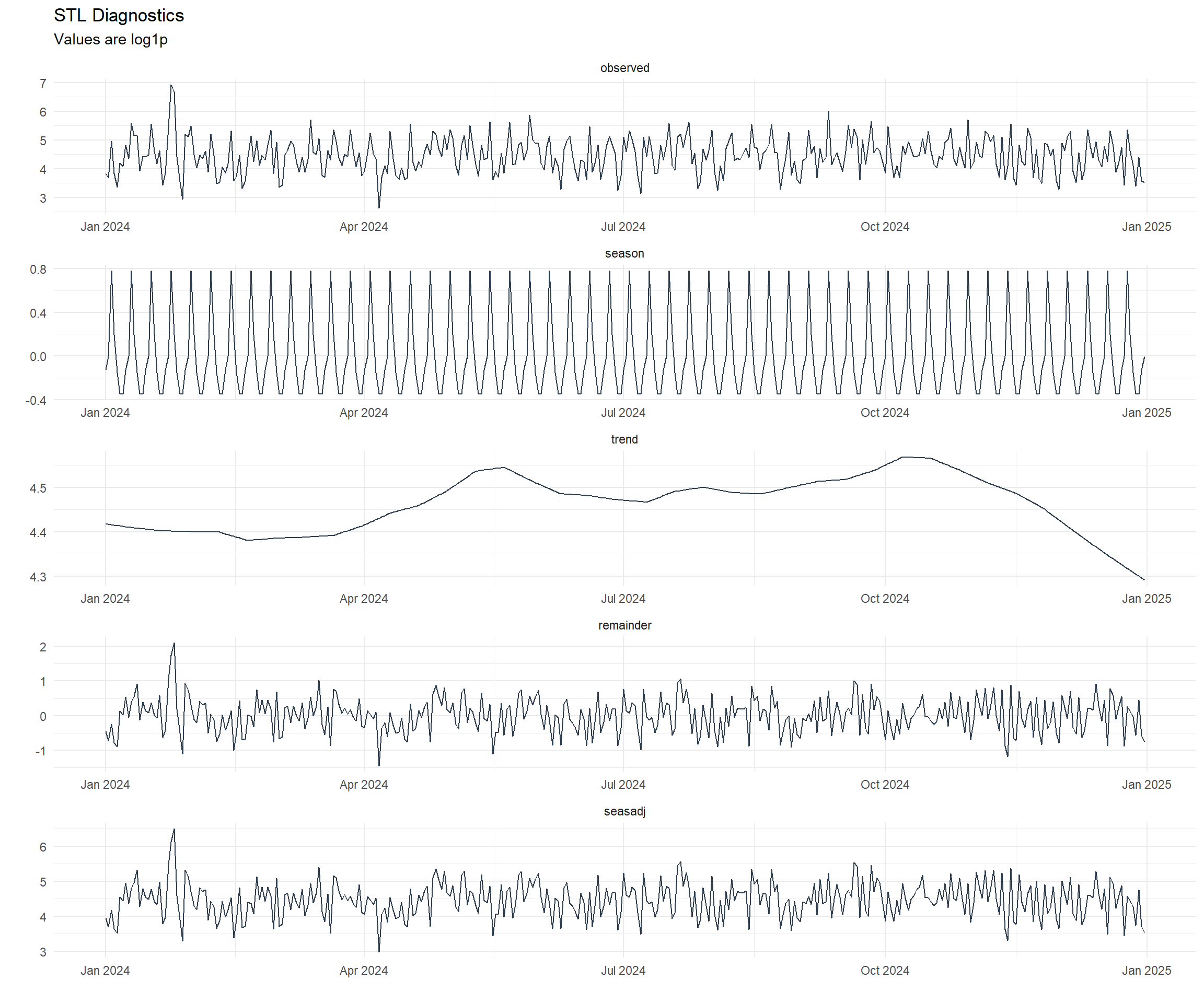# Mapping

So now that we have seen all the downloads in variaous ways, where did they all come from? Lets take a look.

library(tmaptools)
library(countrycode)
library(mapview)
library(htmlwidgets)
library(webshot)

mapping_dataset(.data_year = "2021") %>%
knitr::kable()
country latitude longitude display_name icon
United States 39.783730 -100.445882 United States https://nominatim.openstreetmap.org/ui/mapicons//poi_boundary_administrative.p.20.png
Hong Kong SAR China 22.350627 114.184916 香港 Hong Kong, 中国 https://nominatim.openstreetmap.org/ui/mapicons//poi_boundary_administrative.p.20.png
# l <- map_leaflet(.data = data_tbl)
# mapshot(x = l, file = "map.png")

l <- map_leaflet()
try(webshot("downloads_map.html", file = "map.png", cliprect = "viewport"))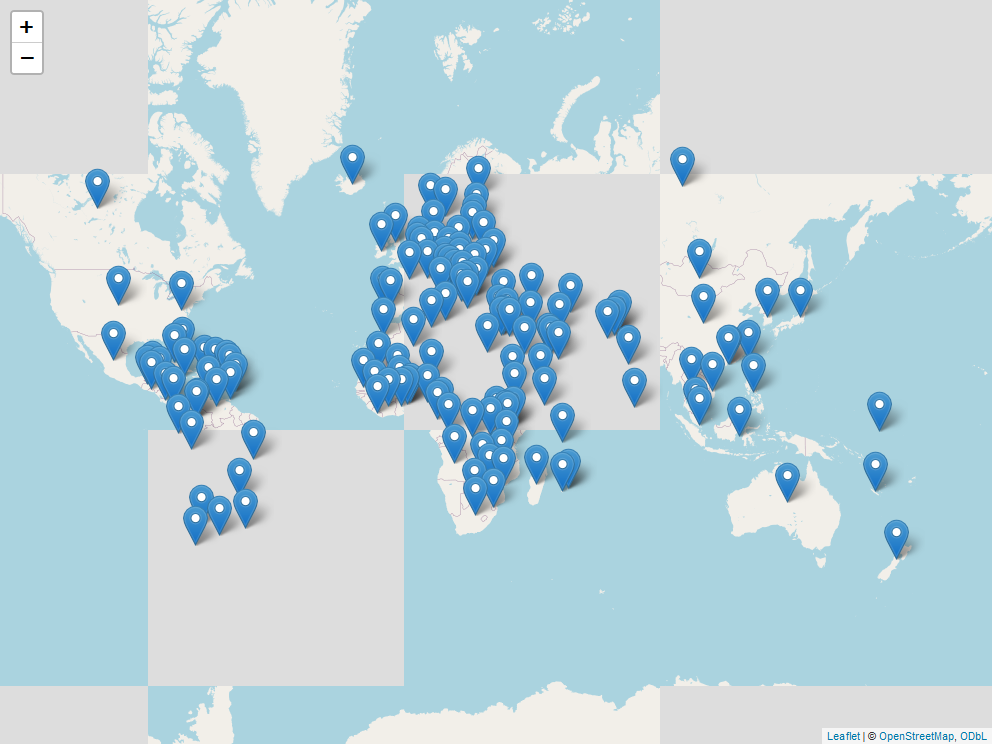There was a total of 121 different countries that downloaded healthyverse packages in 2021.

# GitHub Stats

Now lets take a look at some quick stats from my GitHub

First my contribution grid: